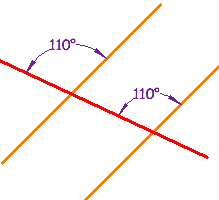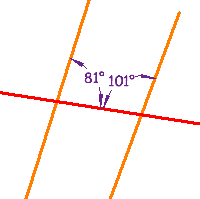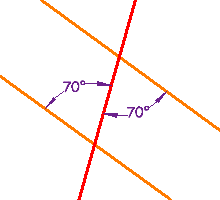# Parallel Lines, and Pairs of Angles

## Parallel Lines

Lines are parallel if they are always the same distance apart (called "equidistant"), and will never meet. Just remember:

### Always the same distance apart and never touching.

The red line is parallel to the blue line in each of these examples:Example 1 Example 2

Parallel lines also point in the same direction.

Parallel lines have so much in common. It's a shame they will never meet!

## Pairs of AnglesWhen parallel lines get crossed by another line (which is called a Transversal), you can see that many angles are the same, as in this example:

These angles can be made into pairs of angles which have special names.

Click on each name to see it highlighted:

Now play with it here. Try dragging the points, and choosing different angle types. You can also turn "Parallel" off or on:

## Testing for Parallel Lines

Some of those special pairs of angles can be used to test if lines really are parallel:

 If Any Pair Of ... Example: Corresponding Angles are equal a = e or Alternate Interior Angles are equal c = f or Alternate Exterior Angles are equal b = g or Consecutive Interior Angles add up to 180° d + f = 180° ... then the lines are Parallel## Examples

 These lines are parallel, because a pair of Corresponding Angles are equal.These lines are not parallel, because a pair of Consecutive Interior Angles do not add up to 180° (81° + 101° =182°) These lines are parallel, because a pair of Alternate Interior Angles are equal